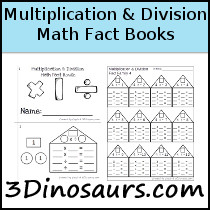Multiplication & Division Math Fact BooksPlease print off as many or as few as you would like as many times as you would like. If you find any errors or typos please let me know. Download is below.

You can buy the full set on Teachers Pay Teachers or Teachers Notebook for \$4.50.

Graphics by Melonheadz Illustrating and Doodles

Multiplication & Division Math Fact Books Sample contains the following:

• Multiplication & Division Math Fact Number 1
• 9 pages of printables

Looking for additional ideas check out my Pinterest board.What Is In The Multiplication & Division Math Fact Books Selling Set

Multiplication & Division Math Fact Books Selling Set contains 316 pages:

• Math facts for Multiplication & Division from 1 to 12
• 12 Beginner Multiplication & Division Math Fact Books
• Single Print and Teacher Print Options for the Math Fact Books
• 15 pages for each begginner books
• 3 pages Types for the Review Multiplication & Division Math Fact Books
• Blank
• 1 number of the number bond filled out
• 2 numbers of the number bond filled out
• Option to use as a No-Prep Printable for the Review Multiplication & Division Math Fact Books

You can buy the full set on Teachers Pay Teachers or Teachers Notebook for \$4.50.

Looking for additional ideas check out my Pinterest board.For help with pdf problems please read this post: Printables Help and Errors.

﻿